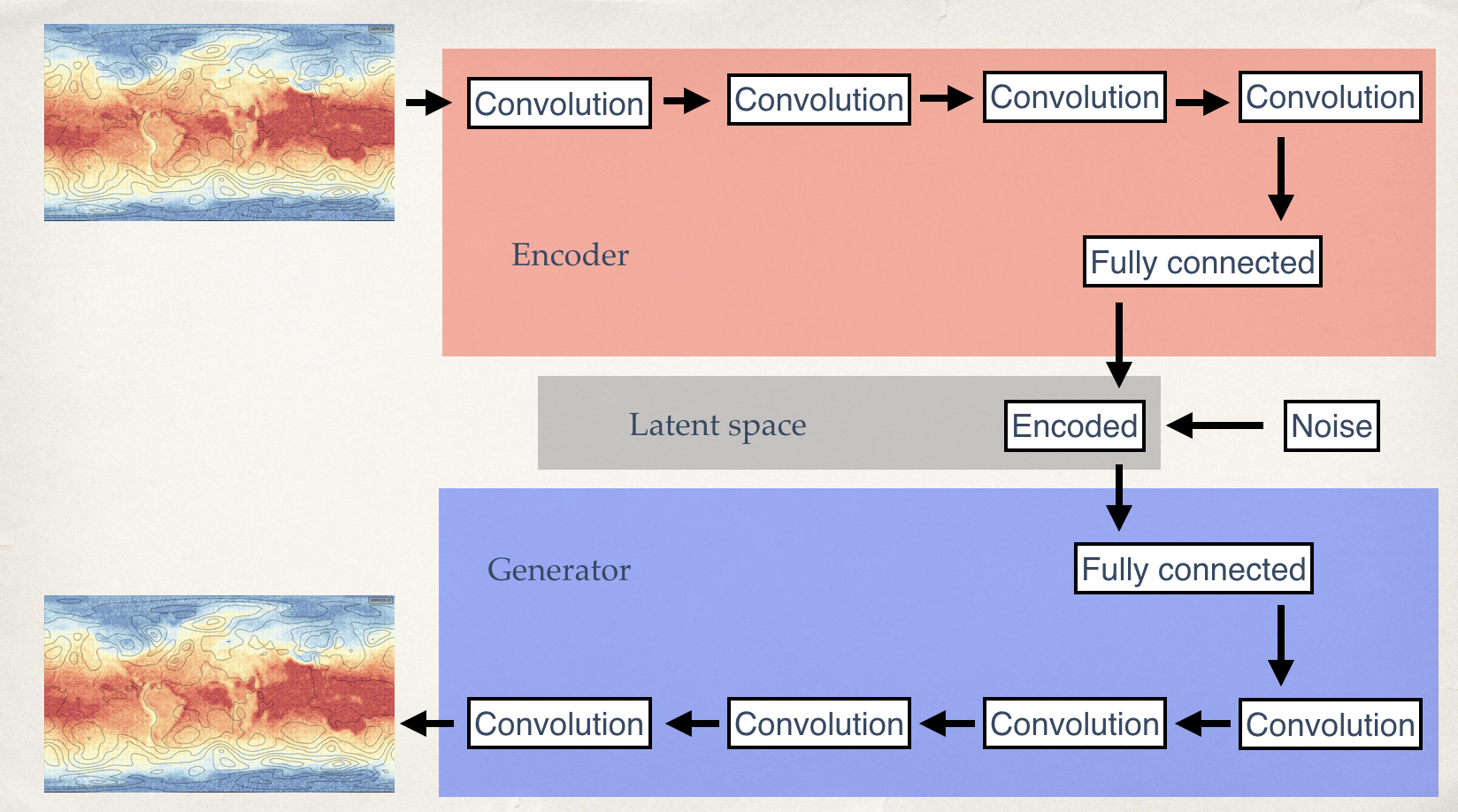# A variational autoencoder for 20CR¶

An autoencoder is a pair of neural nets: one of them (the encoder) compresses an input field into a low-dimensional ‘latent space’, and the other (the generator or decoder) expands the small latent space representation back into the input field. They are trained as a pair - optimising to make `generator(encoder(input))` as close to the original `input` as possible. Effectively an autoencoder learns an effective compressed representation of the original data.

A variational autoencoder is an autoencoder where the generator network can be used independently of the encoder to generate new states similar to the original inputs. This means constraining the latent space to be continuous (two close points in the latent space should produce two similar states when decoded), and complete (any point sampled from the latent space should give ‘meaningful’ content once decoded).

After some experimentation I chose an autoencoder with eight convolutional layers, encoding the features as a 100-dimensional latent space. I failed to get the traditional variational autoencoder design to work, so I adopted a simpler approach: regularising the latent space by explicitly forcing it to have zero mean and unit standard deviation, and perturbing it with gaussian noise.Model structure for the variational autoencoder (Source code).

The autoencoder is specified as five models:

• An encoder
• A generator
• A noise model
• An autoencoder = generator(encoder())
• A variational autoencoder = generator(noise(encoder()))

Then training the variational autoencoder model sets the weights in the encoder and the generator, while maintaining a regularised latent space, and we can deploy the autoencoder (without noise) and generator models using those weights.

To test the model, run the autoencoder on 20CRv2c fields not used in the training process:Validation of the autoencoder. Top panel: T2m, mslp, u and v winds in the original 20CRv3 (at one point in time). Botom panel: same, but after autoencoding. The four scatter-plots compare orignal and encoded values for the four variables. (Validation source code).